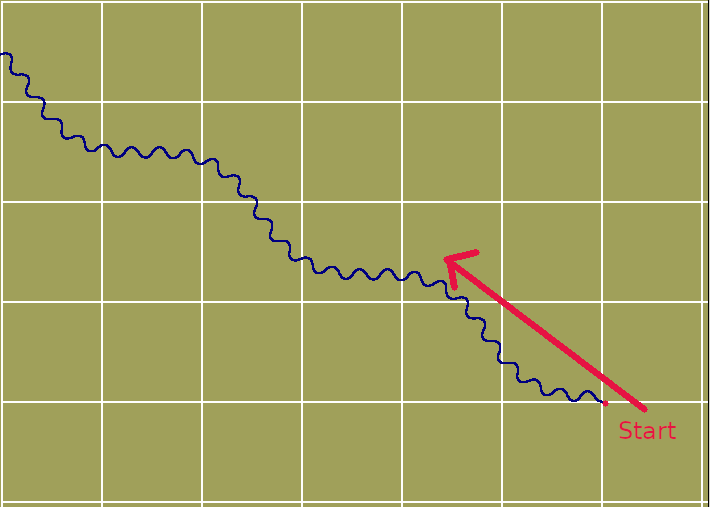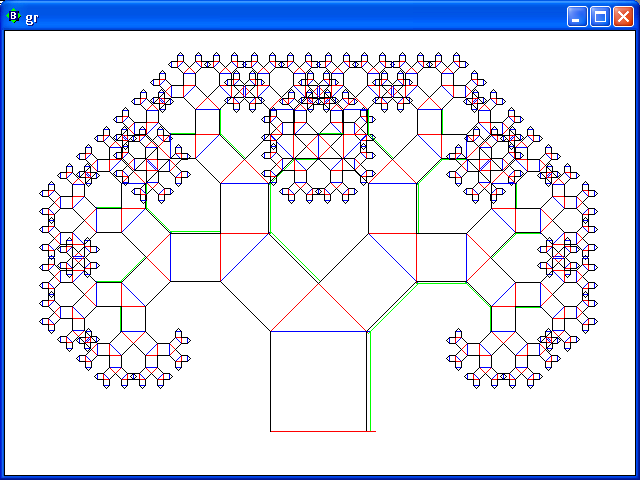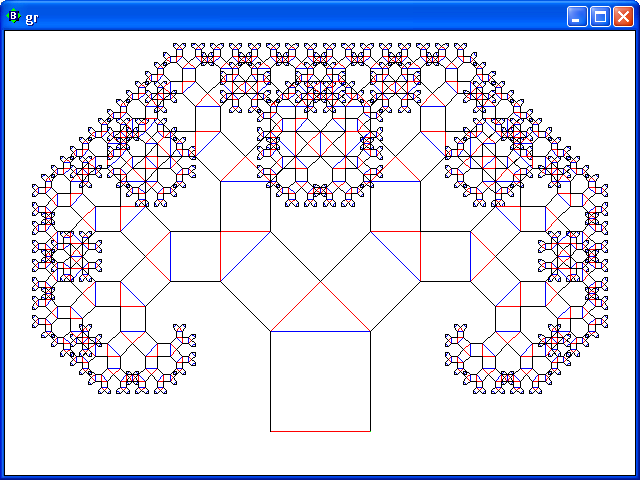# Serendipitous Turtles!

In a moment of madness I wondered if I could create a sine wave which was modulated at a higher frequency- but transverse to its current direction ( imagine bending a wire into a whole lot of small sin waves, then bending that wire bigger amounts but of much longer wavelength.) I thought turtle graphics would help.

Here's my first attempt- I got it wrong!!More accurate turtle graphics WOULD have helped. But LB's native commands can't be used for accurate graphics since they round off distances and angles.

Luckily I wrote replacements long ago- so the errors don't accumulate as in the first attempt. Long time LB user. See my website! diga.me.uk

Basically, here's a warning to LB-ers not to use inbuilt turtle commands for accurate results. It stores nearest screen position and/or an integer angle, so each move/draw can be wrong by up to half a pixel. The next move then starts from the wrong position/angle, and in figures with finer detail than a large square it goes badly and unpredictably wrong. Example is my Koch curve example.. see rosettacode.org/wiki/Koch_curve

My replacements for draw and turning store x, y and orientation as floats, so errors should not exceed one pixel.```    nomainwin

'   Demonstrates turtle drawn directly, avoiding LB's turtle errors and giving
global  pi,             TX,         TY,         Ttheta
pi =4 *atn( 1): TX =102:    TY =252:    Ttheta =0 '   screen left, centred vertically, pointing west

nomainwin

WindowWidth  =1100: WindowHeight =700

open "Demo Koch Snowflake" for graphics_nsb as #wg

#wg "trapclose quit"
#wg "backcolor white"
#wg "goto 2 2 ; down ; box 1202 702 ; up"

call graticule

l =160

for d =0 to 5
TX      = 180 *d +26    '   <<<< start position, direction of each Koch curve.
TY      =  87 *d +51
Ttheta  =0

for side =1 to 3
call fwd l, d
call turn 120
next side

#wg "flush"

next d

#wg "flush"
'#wg "print 1110"

wait

sub quit h\$
call save\$
close #h\$
end
end sub

sub fwd length, depth
scan
if depth <= 0 then
call forward length
else
call fwd length /3, depth -1:     call turn -60
call fwd length /3, depth -1:     call turn 120
call fwd length /3, depth -1:     call turn -60
call fwd length /3, depth -1
end if

end sub

end function

end function

'sub draw lifted, x, y
'    if lifted =0 then #wg "up" else #wg "down"
'    #wg "line "; TX; " "; TY; " "; x; " "; y
'    Ttheta  =atan2( x -TX, TY -y) *180 /pi  '   NB DEGREES.
'    TX      =x
'    TY      =y
'end sub

sub turn angle  '   increment/update global turtle direction ( in DEGREES)
Ttheta =( Ttheta +angle) 'mod 360
end sub

sub forward s
#wg "down ; line "; TX; " "; TY; " "; TX +dx; " "; TY +dy; " ; up"
TX  =TX +dx
TY  =TY +dy
end sub

function atan2( x, y)
Result\$ = "Undetermined"
If ( x = 0) and ( y > 0) Then atan2 = pi / 2:     Result\$ = "Determined"
If ( x = 0) and ( y < 0) Then atan2 = 3 * pi / 2: Result\$ = "Determined"
If ( x > 0) and ( y = 0) Then atan2 = 0:          Result\$ = "Determined"
If ( x < 0) and ( y = 0) Then atan2 = pi:         Result\$ = "Determined"
If Result\$ = "Determined" Then [End.of.function]

BaseAngle = Atn( abs( y) /abs( x))
If (x > 0) and (y > 0) Then atan2 =        BaseAngle
If (x < 0) and (y > 0) Then atan2 = pi    -BaseAngle
If (x < 0) and (y < 0) Then atan2 = pi    +BaseAngle
If (x > 0) and (y < 0) Then atan2 = 2*pi  -BaseAngle
[End.of.function]
end function

sub graticule
#wg "down"
for x =0 to 1500 step 50    '   draw vertical graticule lines
'if x =( Tx -2) then #wg "size 4 ; color red" else #wg "size 2 ; color white"
#wg "line "; x +2; " "; 2; " "; x +2;   " "; 702
next x
for y =0 to 800 step 50
#wg "line "; 2; " "; y +2; " "; 1202; " "; y +2
next y
#wg "up"
end sub

sub saveS
#wg      "flush"
#wg      "getbmp scr 0 0 1110 710"
filedialog "Save as ", "*.bmp", fn\$
bmpsave    "scr", fn\$
end sub
```

Anatoly ( tsh3) commented- 'I remember having troubles drawing Pythagoras tree with turtle So I found it and then fixed with John's code.

Change..

```    #gr "turn -90"
#gr "go ";l
#gr "turn 45"
```
-- .. to ..
```    call turn -90
call forward l
call turn 45
```

So a recursive figure now renders correctly.--```   'some recursive drawing
global  minLength
minLength = 5
nomainwin
WindowWidth = 640
WindowHeight = 480
UpperLeftX = (DisplayWidth - WindowWidth )/2
UpperLeftY = (DisplayHeight -  WindowHeight )/2

open "gr" for graphics_nsb as #gr
#gr "trapclose [quit]"
#gr "home ; down ; posxy midX midY"
width = 2*midX : height = 2*midY

l = 100
#gr "place ";midX-l/2;" ";400
#gr "turn 90"
'    print l
call box l
#gr "color red; go 10"
wait

sub box l
'recursion exit condition
scan
if l < minLength then
'it should left in same position as if drawn
#gr "go ";l
exit sub
end if
'draw a box
for i = 1 to 4
#gr "color ";word\$("red green blue black",i)
#gr "go ";l
#gr "turn -90"
next
'set place for a next box
#gr "turn -90"
#gr "go ";l
#gr "turn 45"
'call recursively
call box l/sqr(2)
'goto next position
#gr "turn 90"
'call recursively
call box l/sqr(2)
'now set to back position...
#gr "turn 45"
#gr "go ";l
#gr "turn -90"
end sub

[quit]
close #gr
end

fixed code
Copy Code
'some recursive drawing
'+Turtle fix by Tenochtitlanuk
'   Demonstrates turtle drawn directly, avoiding LB's turtle errors and giving
global  pi,             TX,         TY,         Ttheta
pi =4 *atn( 1): TX =102:    TY =252:    Ttheta =0 '   screen left, centred vertically, pointing west
' // ---------------------------------
global  minLength
minLength = 4
nomainwin
WindowWidth = 640
WindowHeight = 480
UpperLeftX = (DisplayWidth - WindowWidth )/2
UpperLeftY = (DisplayHeight -  WindowHeight )/2

open "gr" for graphics_nsb as #gr
#gr "trapclose [quit]"
#gr "home ; down ; posxy midX midY"
width = 2*midX : height = 2*midY

l = 100
TX =midX-l/2:    TY =400
'    print l
call box l
#gr "color red; go 10"
wait

sub box l
'recursion exit condition
scan
if l < minLength then
'it should left in same position as if drawn
call forward l
exit sub
end if
'draw a box
for i = 1 to 4
#gr "color ";word\$("red green blue black",i)
call forward l
call turn -90
next
'set place for a next box
call turn -90
call forward l
call turn 45
'call recursively
call box l/sqr(2)
'goto next position
call turn 90
'call recursively
call box l/sqr(2)
'now set to back position...
call turn 45
call forward l
call turn -90
end sub

[quit]
close #gr
end

'-------------------------------------------------
'Turtle fix by Tenochtitlanuk, with globals above
sub turn angle  '   increment/update global turtle direction ( in DEGREES)
Ttheta =( Ttheta +angle) 'mod 360
end sub

sub forward s
#gr "down ; line "; TX; " "; TY; " "; TX +dx; " "; TY +dy; " ; up"
TX  =TX +dx
TY  =TY +dy
end sub